# BiLevel-based DOE on the Sobieski SSBJ test case¶

from __future__ import division, unicode_literals

from copy import deepcopy
from os import name as os_name

from gemseo.api import configure_logger, create_discipline, create_scenario
from gemseo.problems.sobieski.core import SobieskiProblem

IS_NT = os_name == "nt"

configure_logger()


Out:

<RootLogger root (INFO)>


## Instantiate the disciplines¶

First, we instantiate the four disciplines of the use case: SobieskiPropulsion, SobieskiAerodynamics, SobieskiMission and SobieskiStructure.

propu, aero, mission, struct = create_discipline(
[
"SobieskiPropulsion",
"SobieskiAerodynamics",
"SobieskiMission",
"SobieskiStructure",
]
)


## Build, execute and post-process the scenario¶

Then, we build the scenario which links the disciplines with the formulation and the optimization algorithm. Here, we use the BiLevel formulation. We tell the scenario to minimize -y_4 instead of minimizing y_4 (range), which is the default option.

We need to define the design space.

design_space = SobieskiProblem().read_design_space()


Then, we build a sub-scenario for each strongly coupled disciplines, using the following algorithm, maximum number of iterations and algorithm options:

algo_options = {
"xtol_rel": 1e-7,
"xtol_abs": 1e-7,
"ftol_rel": 1e-7,
"ftol_abs": 1e-7,
"ineq_tolerance": 1e-4,
}
sub_sc_opts = {"max_iter": 30, "algo": "SLSQP", "algo_options": algo_options}


### Build a sub-scenario for Propulsion¶

This sub-scenario will minimize SFC.

sc_prop = create_scenario(
propu,
"DisciplinaryOpt",
"y_34",
design_space=deepcopy(design_space).filter("x_3"),
name="PropulsionScenario",
)


### Build a sub-scenario for Aerodynamics¶

This sub-scenario will minimize L/D.

sc_aero = create_scenario(
aero,
"DisciplinaryOpt",
"y_24",
deepcopy(design_space).filter("x_2"),
name="AerodynamicsScenario",
maximize_objective=True,
)


### Build a sub-scenario for Structure¶

This sub-scenario will maximize log(aircraft total weight / (aircraft total weight - fuel weight)).

sc_str = create_scenario(
struct,
"DisciplinaryOpt",
"y_11",
deepcopy(design_space).filter("x_1"),
name="StructureScenario",
maximize_objective=True,
)


### Build a scenario for Mission¶

This scenario is based on the three previous sub-scenarios and on the Mission and aims to maximize the range (Breguet).

sub_disciplines = [sc_prop, sc_aero, sc_str] + [mission]
design_space = deepcopy(design_space).filter("x_shared")
system_scenario = create_scenario(
sub_disciplines,
"BiLevel",
"y_4",
design_space,
parallel_scenarios=False,
reset_x0_before_opt=True,
scenario_type="DOE",
)


Note

Setting reset_x0_before_opt=True is mandatory when doing a DOE in parallel. If we want reproducible results, don’t reuse previous xopt.

system_scenario.formulation.mda1.warm_start = False
system_scenario.formulation.mda2.warm_start = False


Note

This is mandatory when doing a DOE in parallel if we want always exactly the same results, don’t warm start mda1 to have exactly the same process whatever the execution order and process dispatch.

for sub_sc in sub_disciplines[0:3]:
sub_sc.default_inputs = {"max_iter": 20, "algo": "L-BFGS-B"}

n_processes = 4
if IS_NT:  # Under windows, dont do multiprocessing
n_processes = 1

run_inputs = {
"n_samples": 30,
"algo": "lhs",
"algo_options": {"n_processes": n_processes},
}

system_scenario.execute(run_inputs)

system_scenario.print_execution_metrics()


### Plot the optimization history view¶

system_scenario.post_process("OptHistoryView", show=True, save=False)

•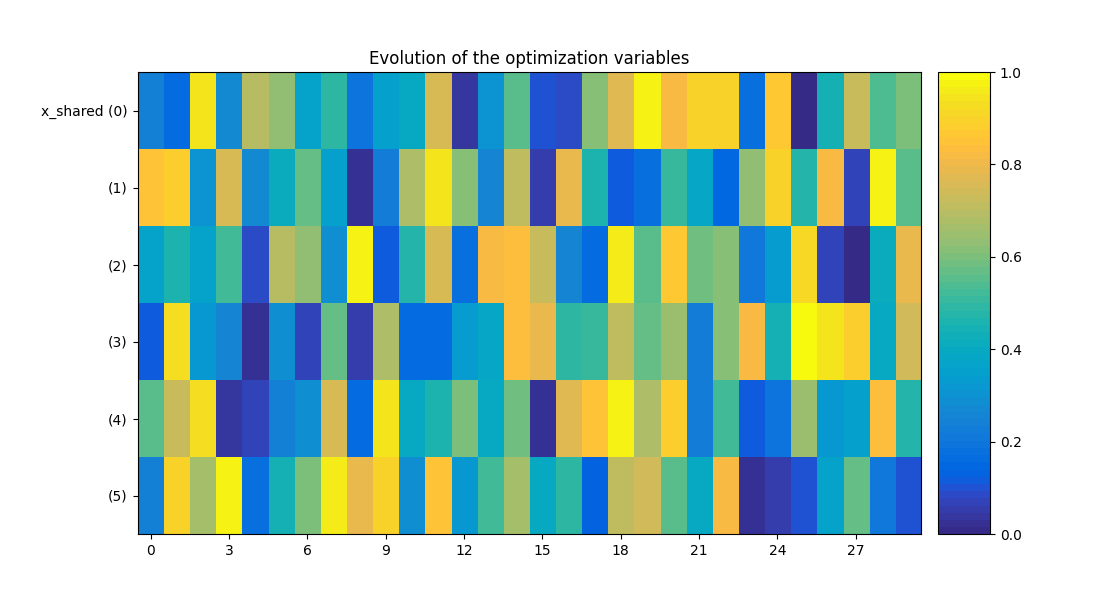•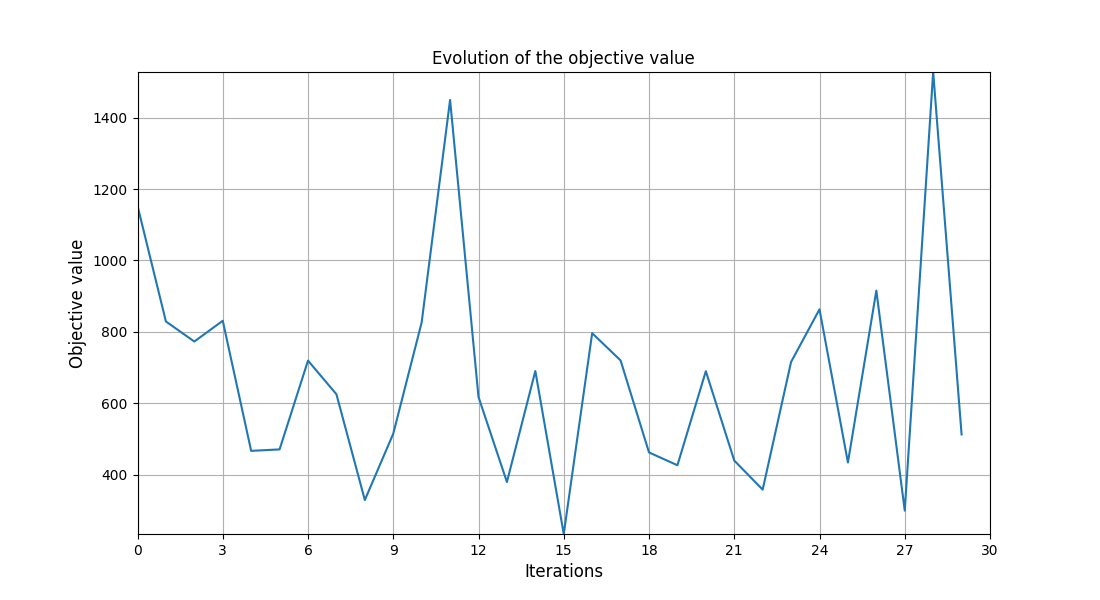•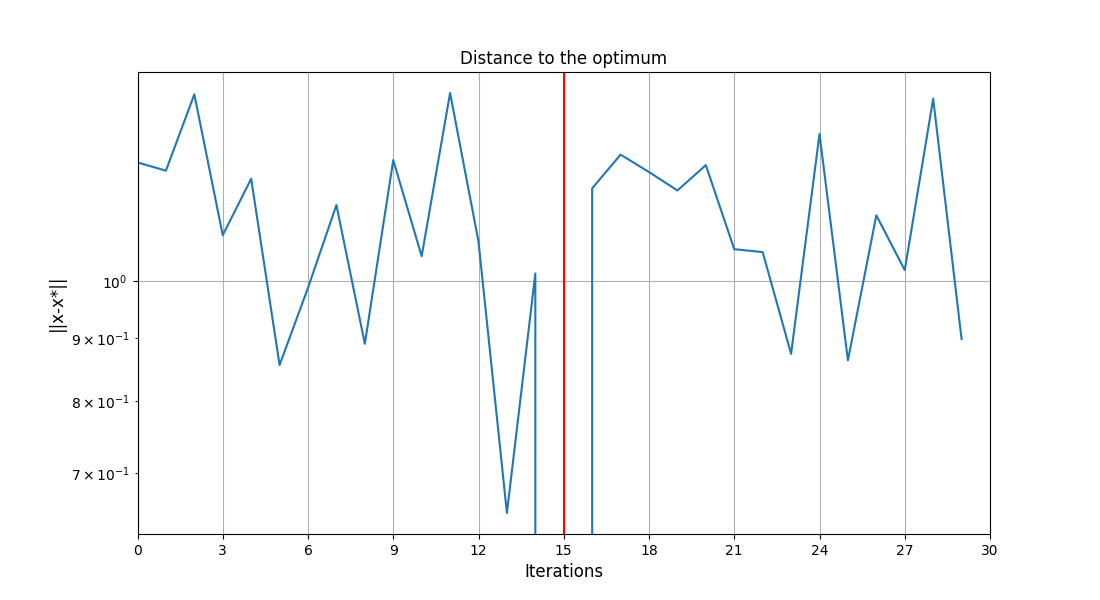Out:

/home/docs/checkouts/readthedocs.org/user_builds/gemseo/conda/3.0.3/lib/python3.8/site-packages/gemseo/post/opt_history_view.py:312: UserWarning: FixedFormatter should only be used together with FixedLocator
ax1.set_yticklabels(y_labels)

<gemseo.post.opt_history_view.OptHistoryView object at 0x7fc29e235d90>


### Plot the scatter matrix¶

system_scenario.post_process(
"ScatterPlotMatrix", show=True, save=False, variables_list=["y_4", "x_shared"]
)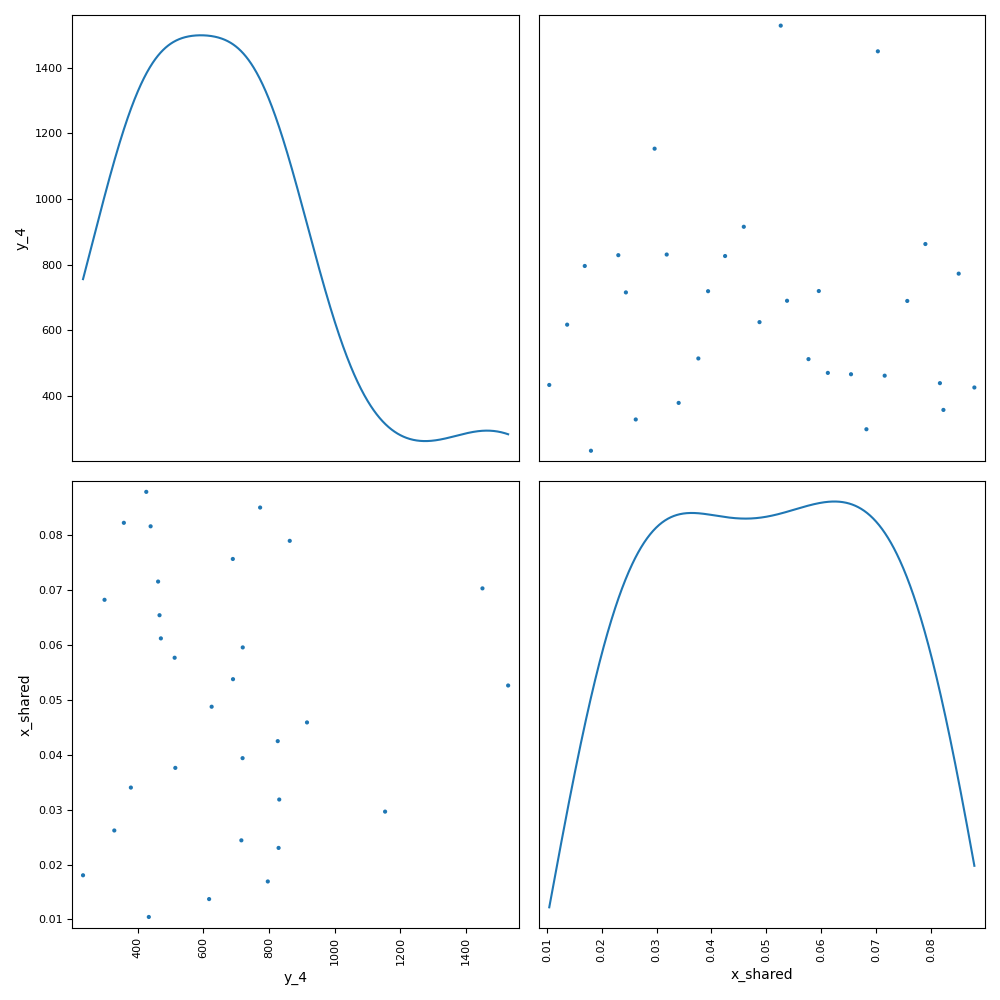Out:

<gemseo.post.scatter_mat.ScatterPlotMatrix object at 0x7fc29dd6b250>


### Plot parallel coordinates¶

system_scenario.post_process("ParallelCoordinates", show=True, save=False)

•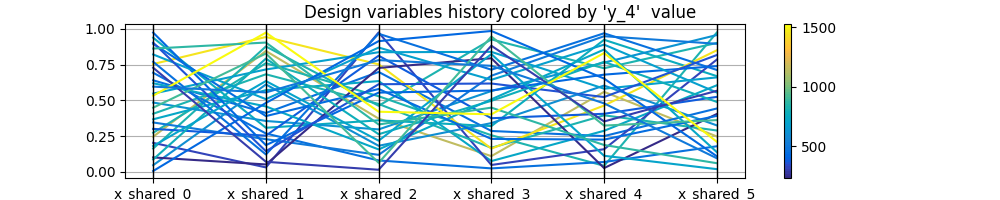•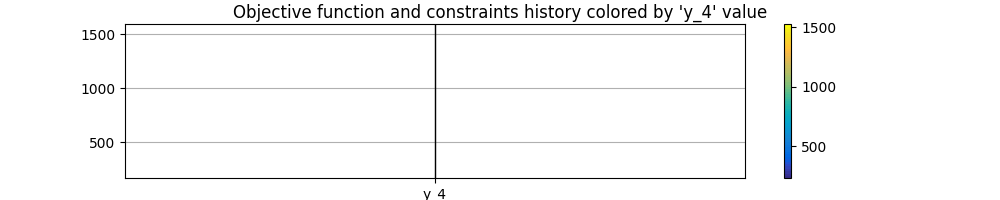Out:

<gemseo.post.para_coord.ParallelCoordinates object at 0x7fc29aee5dc0>


### Plot correlations¶

system_scenario.post_process("Correlations", show=True, save=False)


Out:

<gemseo.post.correlations.Correlations object at 0x7fc29b10a070>


Total running time of the script: ( 0 minutes 6.147 seconds)

Gallery generated by Sphinx-Gallery